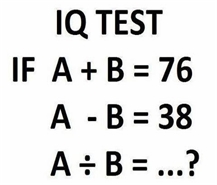﻿ Number Puzzles with Answers | Number Puzzles for Interviews | Number Puzzles Questions Answers 2013 | Number Puzzles Quiz Questions and Answers Pdf 2013 | Number Series Puzzles Questions Answers | Number Puzzles Interview Questions Answers | Cross Number Puzzles | Number Puzzles for Math Geniuses | Brain Puzzle Questions | Technical Interview Questions and Answers Puzzles | Science Puzzles and Riddles with Answers | Number Puzzles Japanese | Math Number Puzzles with Answers | Puzzles Asked in Interview with Answers Pdf | General Logical Questions Answers | Puzzles Questions and Answers in Hindi | Number Puzzles for Kids | Programming Puzzles Interview Questions | Puzzles Interview Questions Answers Pdf | Puzzles Questions and Answers | Puzzles Ebooks Free Download Pdf | Puzzles and Riddles with Answers | Interview Puzzle Questions|EnggRoom
Online Play Puzzles
Online Play Sudoku
Numbering Puzzles
Missing Letters Puzzles
Logical Puzzles
Placement Paper
Company Placement Paper
Gate Paper
Miscellneous
Best Project Ideas
Interviews Questions For Freshers
Request
Feedback

### Join With Facebook

Puzzle 6:- How Many Squares?Ans:-26.
Sol:-Count Carefully.

Puzzle 7:- What number should replace the question mark?Ans:-9.
Sol:-The number at the centre of each triangle equals the sum of the lower two numbers minus the top number.

Puzzle 8:- What number should replace the question mark?Ans:-3.

Sol:-A+B=76 and A-B=38 then
A=57 And B=19
A/B=57/19=3

Puzzle 9:- What number should replace the question mark?Ans:-1.

Sol:-Multiply the bottom two numbers then take the square root, i.e.
1×4 = 4 and √4 = 2.

Puzzle 10:- What number should replace the question mark?Ans:-10.

Sol:-Each diagonal line of numbers, starting with the top left hand corner number, increases by 1 each time.
Example
4
2+3=5
2+1+3=6
2+1+2+2=7
4+1+3=8
5+4=9
10